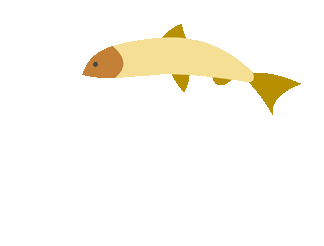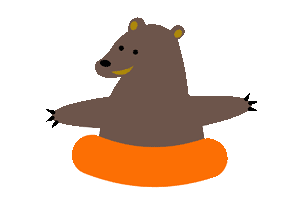top of page## Perimeter and Area

##### of Squares and Rectangles (Resources)
1. Find the Perimeter

Find the perimeter of rectangles.

2. Brain Pop Area

A great movie that explains area in very simple terms.

##### Squares and Rectangles (Games)

Find the perimeter of squares and rectangles and shoot some hoops!

2. Perimeter of Squares and Rectangles Quiz

Scroll down to test your knowledge.

3. Swords

Answer questions correctly and test your aim with a sword against the pirates. This also has surface area questions.

##### of Triangles and Parallelograms (Resources)
1. The Geometry of Triangles

Information on triangles from Cool Math.

2. Properties of Parallelograms

3. Explore Parallelograms

Interactive website for learning about parallelograms.

4. Finding Area of Parallelograms

Cool math shows you how to find the area of parallelograms

##### Triangles and Parallelograms (Games)
1. Identify Types of Triangles Baseball

Classify triangles while playing baseball.

2. Find the Area of Triangles Baseball

Remember the formula and find that area during a game of baseball.

3. Area of Triangles Quiz

Scroll down to test you ability to correctly find the area of triangles.

4. Area of a Triangle in Relationship in Relationship to Rectangles

Vocally guided interactive power point about area.

5. Area of Parallelograms Shape Cutter

Make parallelograms and then cut them to make rectangles or squares, calculate the area and perimeter of the parallelogram.

##### Perimeter Mixed Practice (Resources)
###### Perimeter Mixed Practice (Resources)
1. Help with Perimeter of Different Shapes

Shows the formulas and help find perimeter.

2. Finding Perimeter

Cool Math has lots of information on perimeter.

###### Perimeter Mixed Practice (Games)
1. Shape Explorer

Find the perimeter of the given shape (includes irregular shapes).

##### Area Mixed Practice (Resources)

Area Mixed Review (Resources)

1. Finding Area

Cool Math has lots of information on area.

2. Formulas for Finding Area

Very good notes for helping you with finding the area of shapes.

3. Area Tool

Find the area of parallelograms, trapezoids, and triangles.

4. Areas in Geometry

Find the area of triangles, parallelograms, and rectangles.

5. Area of Polygons

Omit circles, that is a 6th grade skill or after you master the polygons move on to circles.

###### Area Mixed Review (Games)
1. Finding the Area

Timed test to see how well you can find the area of various objects.

##### Perimeter & Area Mixed (Resources)
1. Perimeter and Area

Very cool site for help with perimeter and area.

##### Perimeter & Area Mixed (Games)
1. Review Perimeter and Area

Find perimeter and area and play a game of basketball.

2. Fun Brain Area and Perimeter

Select the level of difficulty and start playing.bottom of page